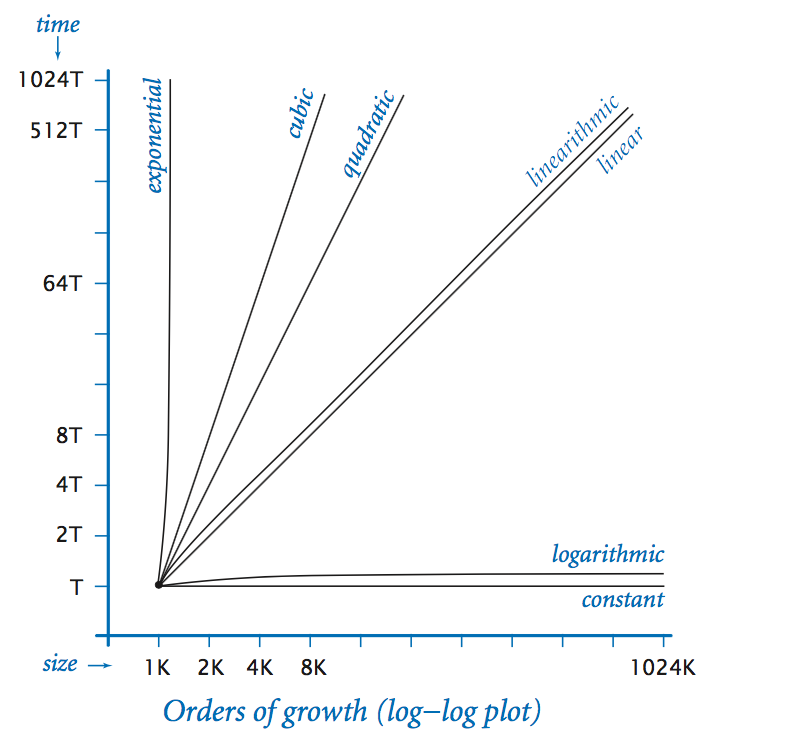# Topics

• Running time
• Experiments
• Observations
• Doubling hypotheses
• The $$\sim$$ notation
• Order of growth
• Orders of growth plot

# Running time

• The cost of software includes the cost of developing, running, and maintaining it. We will focus on the cost of running it only.
• Two main costs
• time
• space (memory)
• Running time is more critical than memory usage and we’ll focus on it.

# Experiments

• To get the running time of a program, we can perform experiments: just run the program and measure its running time using some kind of stopwatch.
• But it's dificult to measure running time exactly due to the way modern computer systems operate. Computers run several (user & system) threads concurrently by interleaving them in time, etc.
• Luckily approximate measurements suffice for our purpose.
• Kotlin provides a standard library called kotlin.system.measureTimeMillis for measuring the time it takes to execute a code block. So running

val time = kotlin.system.measureTimeMillis { /* body of code block */ }
will execute the body of the code block and returns the running time in milliseconds in the variable time.
• Kotlin also provides another library called kotlin.system.measureNanoTime that works exactly the same way as kotlin.system.measureTimeMillis except that it returns the running time in nanoseconds.

# Observations

• Most problems solved by a program has a natural problem size that characterizes the difficulty of the computational task.
• This problem size is either the size of the input or the value of a command-line argument.
• The running time increases with problem size, but the amount and rate of increase depends on the program’s algorithm.
• The running time is relatively insensitive to the input itself; it depends primarily on the problem size.

# Doubling hypotheses

• For many programs, we can formulate a hypothesis for the following question: What is the effect on the running time of doubling the size of the input?
• We can write a test harness that takes some other program and runs it on a range of sizes that increases as the powers of 2.
• By plotting the graph of input size against running time we can get the answer to our doubling hypothesis.

# The $$\sim$$ notation

• Let $$f$$ and $$g$$ be functions on $$\mathbb{N}$$. We write $$\sim f(n)$$ to represent any quantity that, when divided by $$f(n)$$, approaches 1 as $$n$$ grows without bound. We also write $$g\sim f$$ (or $$g(n)\sim f(n)$$) to indicate that $$g(n)/f(n)\to 1$$ as $$n\to\infty$$.
• For example $$n(n-1)(n-2)/6 \sim n^3/6$$.

# Order of growth

• For many programs, the running time $$T(n)$$ of a program on input of size $$n$$ satisfies the relationship $$T(n) \sim c f(n)$$ where $$c$$ is a constant and $$f(n)$$ is a function known as the order of growth of the running time. Typically, $$f(n)$$ is a function such as $$\log n$$, $$n$$, $$n\log n$$, $$n^2$$, $$n^3$$, etc.
• Common orders-of-growth:
description function factor for doubling hypothesis
constant 1 1
logarithmic log n 1
linear n 2
linearithmic n log n 2